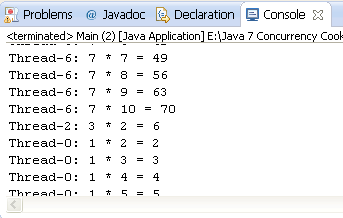# 线程管理之线程创建和运行解析Java提供两种方式创建线程：

1.     创建一个名为Calculator的类，这个类要实现Runnable接口。

 `1` `public` `class` `Calculator ``implements` ```Runnable { ```

2.   声明一个名为number 的private int为属性，然后实现构造函数并初始化其值。

 `1` `private` `int` `number;`
 `2` `public` `Calculator(``int` ```number) { ```
 `3` `    ``this``.number=number;`
 `4` `}`

3.   实现run()方法. 这方法是给我们创建的线程执行指令，所以这个方法将计算数字乘法表。

 `1` `@Override`
 `2` `public` `void` ```run() { ```
 `3` `  ``for` `(``int` `i=``1``; i<=``10````; i++){ ```
 `4` `   ``System.out.printf(``"%s: %d * %d = %d\n"``,Thread.currentThread().getName(),number,i,i*number);`
 `5` `}`
 `6` `}`

4.   现在, 实现程序的Main类。创建一个名为 Main的类并包含 main() 方法.

 `1` `public` `class` ```Main { ```
 `2` `    ``public` `static` `void` ```main(String[] args) { ```

 `1` `for` `(``int` `i=``1``; i<=``10````; i++){ ```
 `2` `   ``Calculator calculator=``new` `Calculator(i); Thread thread=``new` `Thread(calculator);`
 `3` `   ``thread.start();`
 `4` `}`

6.   运行程序并观察不同的线程是如何并行工作的。``````package com.java.demo;

public class Calculator implements Runnable {
private int number;

public Calculator(int number) {

this.number = number;
}

@Override
public void run() {
for (int i = 1; i <= 10; i++) {
System.out.printf("%s: %d * %d = %d\n", Thread.currentThread().getName(), number, i, i * number);

}
System.exit(0);

}

}
``````
main方法

``````package com.java.demo;

public class Main {

public static void main(String[] args) {
// TODO Auto-generated method stub
Calculator calculator = new Calculator(1);
}

}
``````

1.本站遵循行业规范，任何转载的稿件都会明确标注作者和来源；2.本站的原创文章，请转载时务必注明文章作者和来源，不尊重原创的行为我们将追究责任；3.作者投稿可能会经我们编辑修改或补充。